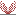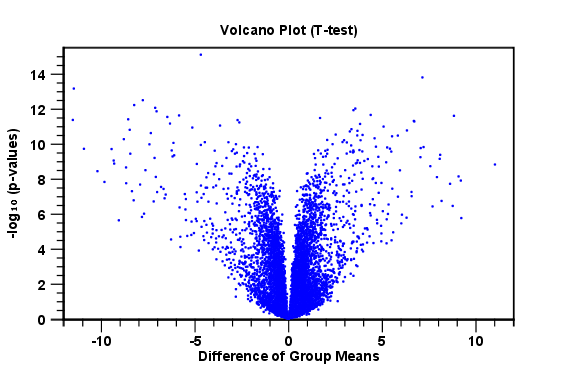## Volcano plots - inspecting the result of the statistical analysis

The results of the statistical analysis are added to the experiment and can be shown in the experiment table. Typically columns containing the differences (or weighted differences) of the mean group values and the fold changes (or weighted fold changes) of the mean group values will be added along with a column of p-values. Also, columns with FDR or Bonferroni corrected p-values will be added if these were calculated. This added information allows features to be sorted and filtered to exclude the ones without sufficient proof of differential expression (learn more in Working with tables).

If you want a more visual approach to the results of the statistical analysis, you can click the Show Volcano Plot () button at the bottom of the experiment table view. In the same way as the scatter plot, the volcano plot is yet another view on the experiment. Because it uses the p-values and mean differences produced by the statistical analysis, the plot is only available once a statistical analysis has been performed on the experiment.

An example of a volcano plot is shown in figure 29.61.Figure 29.61: Volcano plot.

The volcano plot shows the relationship between the p-values of a statistical test and the magnitude of the difference in expression values of the samples in the groups. On the y-axis the -log10 p-values are plotted. For the x-axis you may choose between two sets of values by choosing either 'Fold change' or 'Difference' in the volcano plot side panel's 'Values' part. If you choose 'Fold change' the log of the values in the 'fold change' (or 'Weighted fold change') column for the test will be displayed. If you choose 'Difference' the values in the 'Difference' (or 'Weighted difference') column will be used. Which values you wish to display will depend upon the scale of you data (Read the note on fold change in this section).

The larger the difference in expression of a feature, the more extreme it's point will lie on the X-axis. The more significant the difference, the smaller the p-value and thus the higher the -log10(p) value. Thus, points for features with highly significant differences will lie high in the plot. Features of interest are typically those which change significantly and by a certain magnitude. These are the points in the upper left and upper right hand parts of the volcano plot.

If you have performed different tests or you have an experiment with multiple groups you need to specify for which test and which group comparison you want the volcano plot to be shown. You do this in the 'Test' and 'Values' parts of the volcano plot side panel.

Options for the volcano plot are described in further detail in Plot groups.

If you place your mouse on one of the dots, a small text box will tell the name of the feature. Note that you can zoom in and out on the plot (see Zooming).

In the Side Panel to the right, there is a number of options to adjust the view of the volcano plot. Under Graph preferences, you can adjust the general properties of the volcano plot

• Lock axes This will always show the axes even though the plot is zoomed to a detailed level.
• Frame Shows a frame around the graph.
• Show legends Shows the data legends.
• Tick type Determine whether tick lines should be shown outside or inside the frame.
• Outside
• Inside
• Tick lines at Choosing Major ticks will show a grid behind the graph.
• None
• Major ticks
• Horizontal axis range Sets the range of the horizontal axis (x axis). Enter a value in Min and Max, and press Enter. This will update the view. If you wait a few seconds without pressing Enter, the view will also be updated.
• Vertical axis range Sets the range of the vertical axis (y axis). Enter a value in Min and Max, and press Enter. This will update the view. If you wait a few seconds without pressing Enter, the view will also be updated.

Below the general preferences, you find the Dot properties, where you can adjust coloring and appearance of the dots.

• Dot type
• None
• Cross
• Plus
• Square
• Diamond
• Circle
• Triangle
• Reverse triangle
• Dot
• Dot color. Allows you to choose between many different colors. Click the color box to select a color.

At the very bottom, you find two groups for choosing which values to display:

• Test. In this group, you can select which kind of test you want the volcano plot to be shown for.
• Values. Under Values, you can select which values to plot. If you have multi-group experiments, you can select which groups to compare. You can also select whether to plot Difference or Fold change on the x-axis. Read the note on fold change in this section.

Note that if you wish to use the same settings next time you open a box plot, you need to save the settings of the Side Panel.